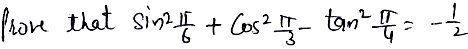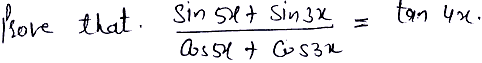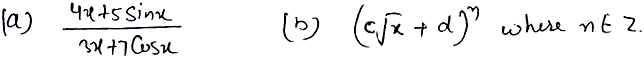Thursday , September 29 2022# 11th Class CBSE Mathematics Compartment Exam 2019-20

## 11th Class CBSE Mathematics Compartment Exam 2019-20

School Name: Himalaya Public School, Sector 13, Rohini, Delhi 110085 India
Time: 3 hours
Maximum Marks: 80
Class: 11th
Date: 14/02/2020
Subject: Mathematics

## General Instructions:

• All questions are compulsory.
• This question paper consists of 36 questions divided into four sections – A, B, C and D.
• Section – A Comprises of 20 question of 1 marks each.
Section – B Comprises of 6 question of 2 marks each.
Section – C Comprises of 6 question of 4 marks each.
Section – D Comprises of 4 question of 6 marks each.
• There is no overall choice. However on internal choice has been Provided in there questions of 1 marks each, two questions of 2 marks each, two questions of 4 marks each and two questions of 6 marks each. You have to attempt only one of the alternative in all such questions.
• Use of calculator is not Permitted.

## 11th Class CBSE Mathematics Compartment: Section A

### Question 1 – Question 10 are Multiple choice type questions. Select the correct option.

#### Question: 1. If A = {1, 2} then the number of elements in P (A) will be

1. 4
2. 2
3. 0
4. None of these

#### Question: 2. If A = {1, 2} and B = {3, 4} then number of subsets that A × B have is

1. 16
2. 4
3. 2
4. None of these

1. ½
2. √3/2
3. -1/2
4. -√3/2

1. 1/13
2. 12/13
3. 1/52
4. 4/5

1. 50
2. 25
3. 5√21
4. 20

1. √3
2. 1/√3
3. – √3
4. -1/√3

1. y² = -24 x
2. x² = 24 y
3. x² = -24 y
4. y² = 24 x

1. 5√3 units
2. 3√5 units
3. 7 units
4. 5 units

1. A – B
2. A – B¹
3. B – A
4. B – A¹

1. Z
2. R
3. W
4. N

### Question 11 to Question 15 (Fill in the blanks)

#### Question: 13.### Q. 16 to 20. Answer the following questions:

#### OR## 11th Mathematics Compartment Exam: Section B

#### Question: 22.## 11th Mathematics Compartment Exam: Section C

#### Question: 32. Find the derivatives of each of the following with respect to x:     [2+2 Marks]## Class XI Computer Science Theory Syllabus (083): Python

Unit No. Unit Name Marks 01. COMPUTER SYSTEMS AND ORGANISATION 10 02. PROGRAMMING AND COMPUTATIONAL …